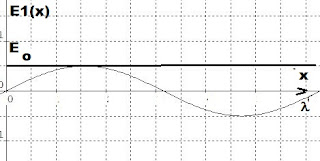## Tuesday, September 7, 2021

### Deriving The Landau Damping Rate Of An E-Field (1)

Analytic  graph for Landau damping- applied to Langmuir waves.

Having seen  how Landau damping scuttles the notion of ATTIP  military -sighted UFOs   as holograms (designed to confuse pilots),  e.g.

It is instructive to see how the Landau damping rate is derivedWe recall here that Landau damping is a result of a solution to the dispersion relation involving  w and k, i.e. the phase velocity resulting is  v f  =  w / k.   We proceed then by considering the effect of a small oscillating (Langmuir) wave associated with  a perturbed plasma distribution  f1(x,v,t) = 0.

Consider then a linear wave of form:

E1 (x’,t) =  E o  sin (kx’ -  w r t)

Where x’ denotes the lab frame of reference.  E o  is a small constant for amplitude.  In the frame of reference x moving with phase speed f  =  w / k >  0 with respect to the laboratory frame the wave field is independent of time t and is given by: E1 (x’,t) =  E o  sin (kx’)   This is represented in the  graphic below.  (E o amplitude position marked by bold horizontal line)Note that all the particles in the background distribution plasma (f o (v)) are affected by this non-time dependent E-field. Some are speeded up, others slowed down.

For simplicity, we focus on only those that have speed v o  in the lab frame at t = 0.  Hence, they have speed  v o  - v f     in the frame moving with the wave phase speed f  =  w / k. (See top graphic.)

Change in energy (d E ) of a given particle:

d E   =   ½ (m v o   +    d v) 2  - ½ m v o2

=   m v o  d v  +  O (d v 2)

where we ignore the term in d 2

Averaging the  energy change over one wavelength:

< d E  >xo     =   m v o  < d v > xo

Since  d v  can be calculated in any frame, we work in the wave frame. Then the change in velocity is:

d v   =    v(t)  -   v o  =  (-e) E / m  òt  o   dt’    sin kx(t) dt’

x(t)  must be along particle orbit so:

x(t’) =   x o  +  òto  v(t”) dt”

Examine again the equation of motion:

mx” =  - e E o sin kx

For which we may write:

mx’ x”  =   - e E o  sin kx (dx/ dt)

d/dt (½ m x’2) =  -e E o/ k [ d/dt (cos kx)]

x’  =       Ö 2 e E o/ km  [cos kx  - cos kx o  ]

Now integrate both sides:

dx/[cos kx  - cos kx o  ] ½  =     Ö (2 e E o/ km)   dt

We  subst.     x   =  x o   +  v o t"'   into integral:

òt’’ o  sin (kx o    +  k v o t"')  dt’"  =

- 1/k v o    [cos (kx o    +  k v o t"') -  cos kx o

And:

v(t")  =   v o   +   E o /ne kv  [cos (kx o    +  k v o t"') -  cos kx o

The preceding  parameter to be subst. into:

x(t')   =   x o   +  òt’ o v (t") dt"

(To be continued)